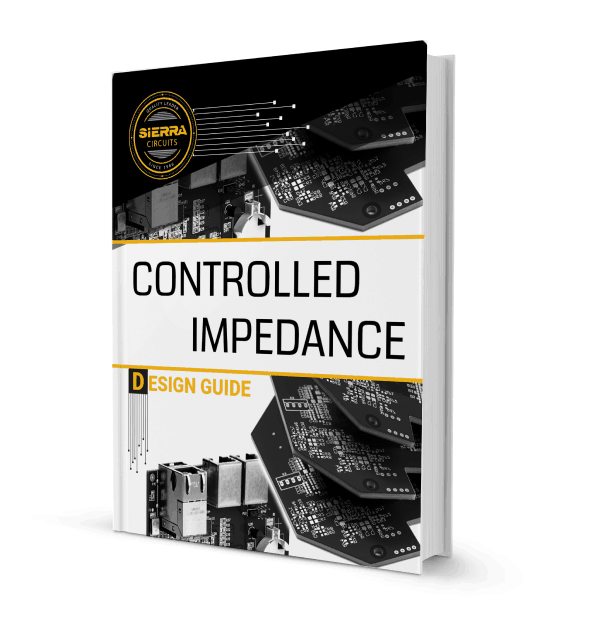## Signal Speed and Propagation Delay in a PCB Transmission Line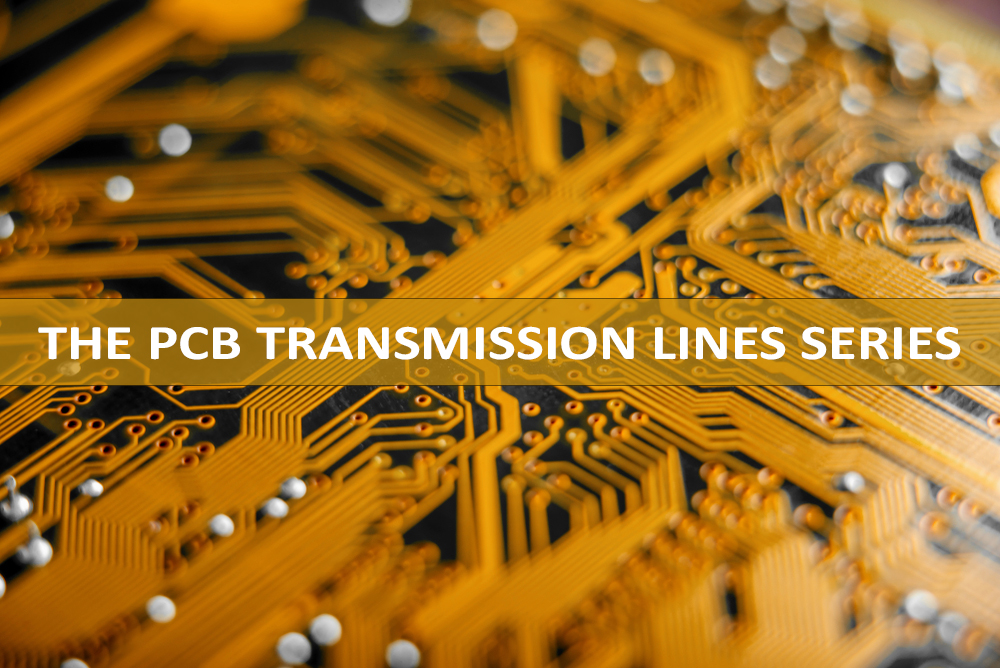Last week, we talked about what a PCB transmission line is. We are now going to focus on the technical side and discuss signal speed and propagation delay, two factors that have a great impact on a transmission line.

At high frequencies, transmission lines need to have a controlled impedance to predict the behavior of the signals and avoid signal reflections, crosstalk, electromagnetic noise, etc. which could damage the signal quality and cause errors.

This is the reason why you need to know at which speed signals propagate on transmission lines and the time they take to do so. We will give you a few equations to calculate the signal speed and the propagation delay for both striplines and microstrips.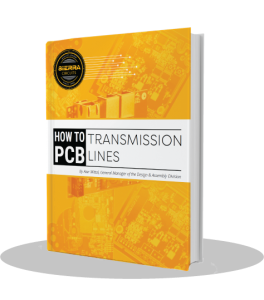Signal speed

Let’s first discuss the speeds at which signals propagate on a PCB interconnect.

Electromagnetic signals travel in vacuum or air at the same speed as of light, which is: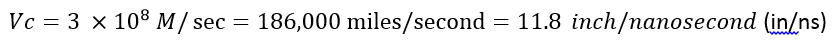A signal travels on a PCB transmission line at a slower speed, affected by the dielectric constant (Er) of the PCB material. The relations for calculating the signal speed on a PCB are given below: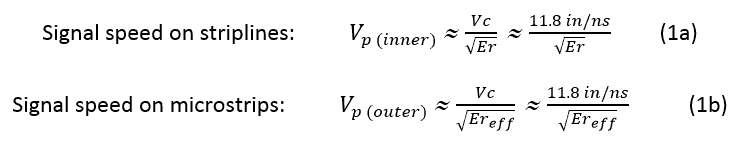Where:
Vc is the velocity of light in vacuum or air
Er is the dielectric constant of the PCB material
Ereff is the effective dielectric constant for microstrips; its value lies between 1 and Er, and is approximately given by: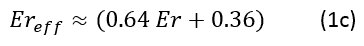Thus, the speeds of signals on a PCB is less than that in air. If Er ≈ 4 (like for FR4 material types), then the speed of signals on a stripline is half that in air, i.e. it is about 6 in/ns.

Henceforth, we can use Vp to denote the speed of signals on a PCB.

Propagation delay (tpd)

The propagation delay is the time taken by a signal to propagate over a unit length of the transmission line: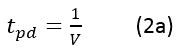Where:
V is the signal speed in the transmission line

In vacuum or air, it equals 85 picoseconds/inch (ps/in).

On PCB transmission lines, the propagation delay is given by: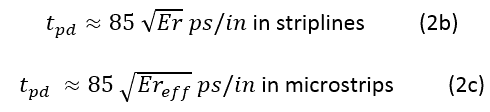The signal speeds and propagation delays for a few PCB materials are given in the table below: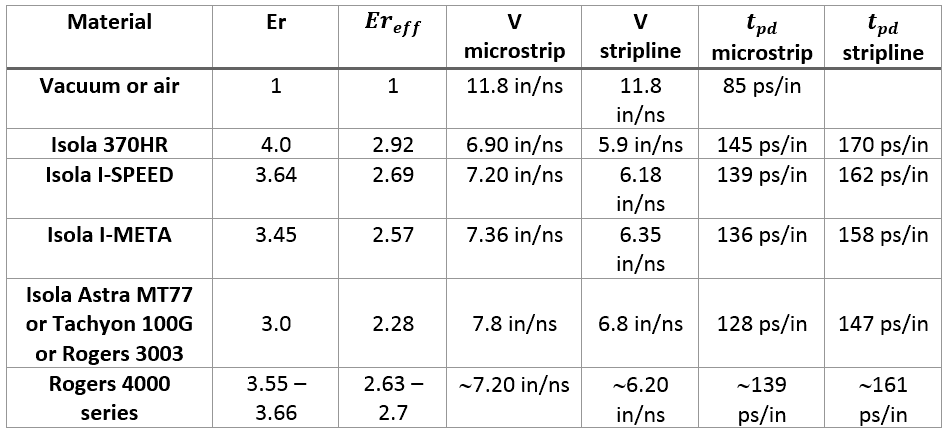Next week, we will give you more math to help you determine whether or not the length of an interconnection has to be considered as a controlled impedance transmission line. We will talk about critical length, shortlines, the rise time from data transfer rate (DTR) or clock frequency, and 3 dB bandwidth.